﻿ Digital Circuits and Systems - Circuits i Sistemes Digitals (CSD) - EETAC - UPCBachelor's Degree in Telecommunications Systems and in Network Engineering1.8. Binary codes and code converters

1.8.1. Number systems. Binary radix 2 (base 2), octal (base 8, hexadecimal (base 16)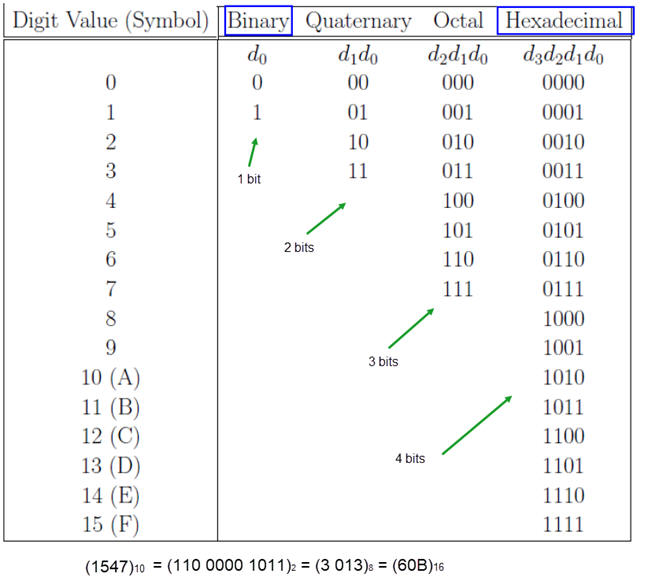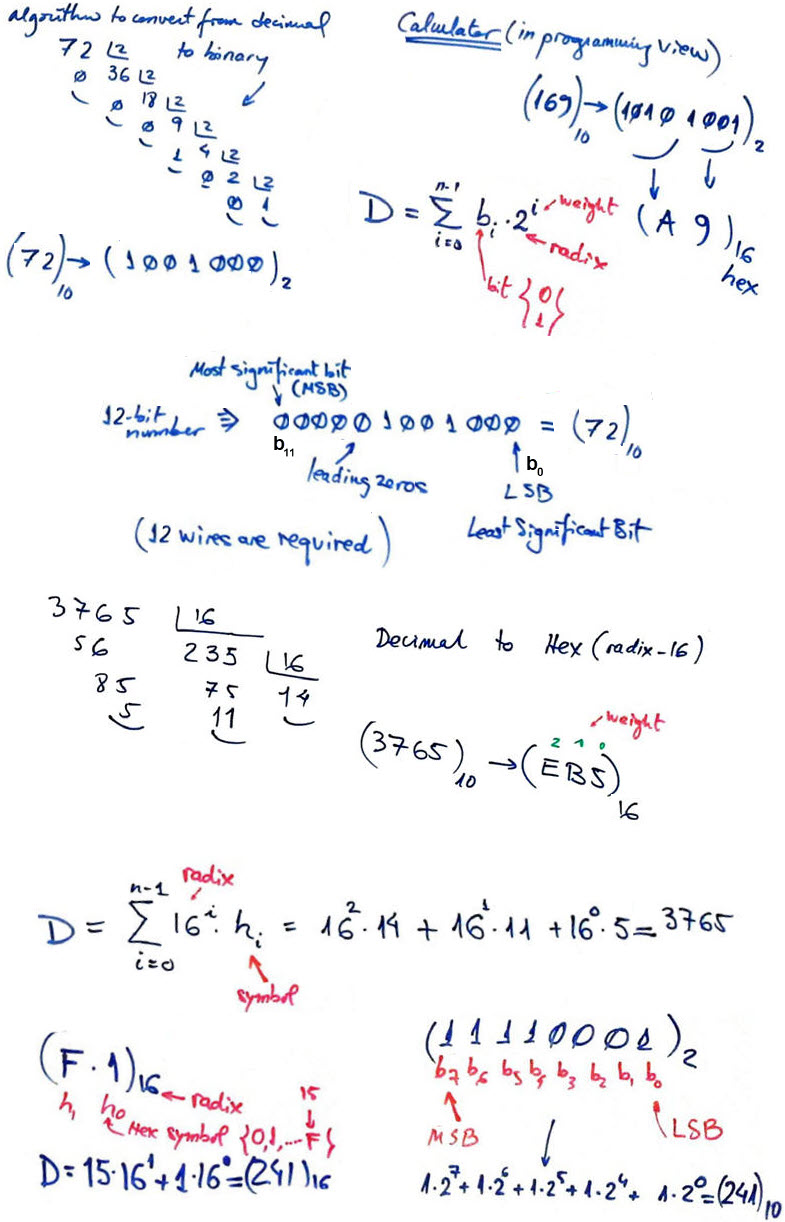1.8.2. Gray code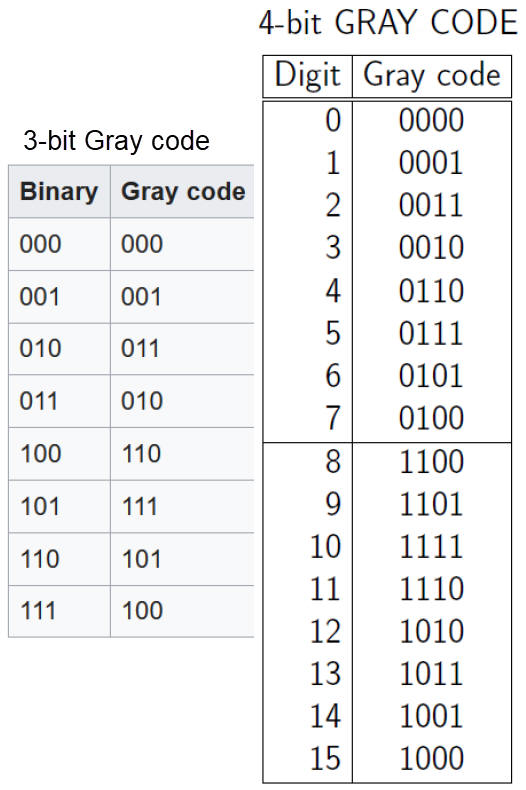1.8.3. BCD (binary-coded decimal)1.8.4. One-hot and one-cold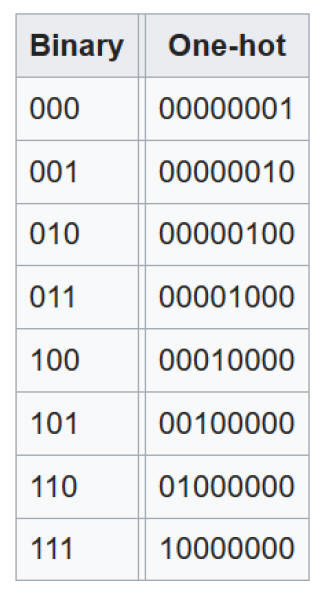1.8.5. Johnson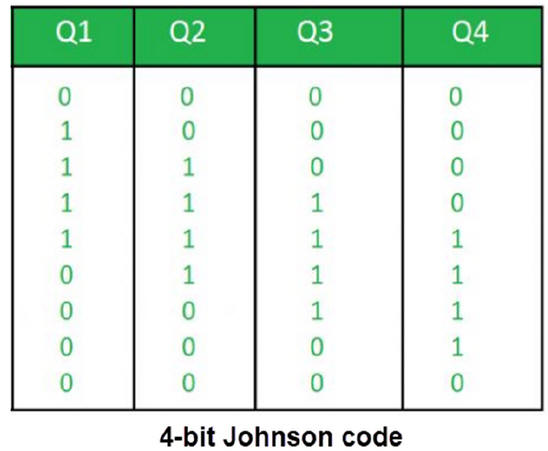1.8.6. Keyboard symbols: ASCII, etc.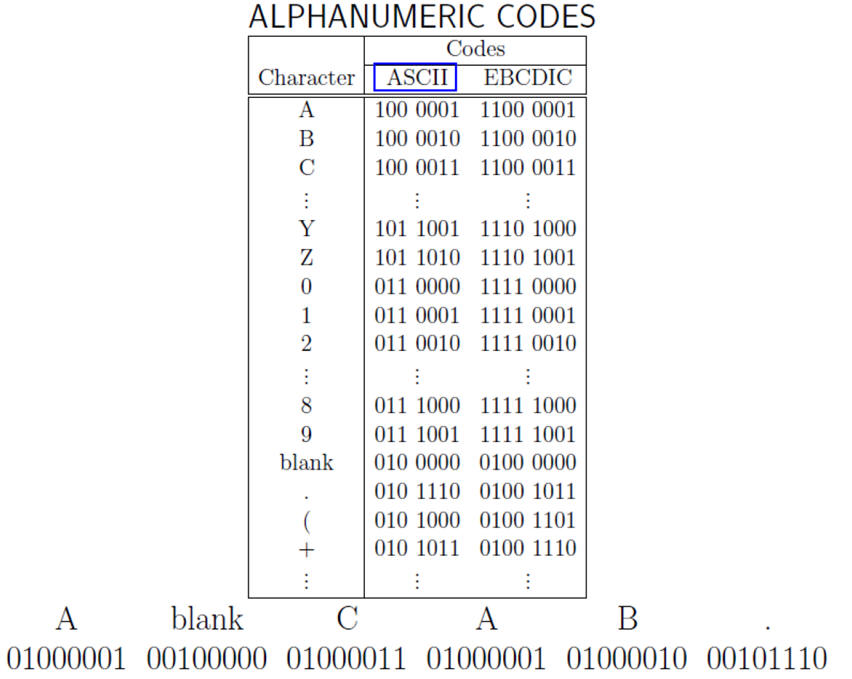1.8.7. Code converter circuits

1.8.7.1. Bin_BCD_converter_6bit

1.8.7.2. Gray_Bin_converter_4bit

1.9. Binary (radix-2, base-2) arithmetic circuits

Binary numbers, symbols, range and how to perform additions.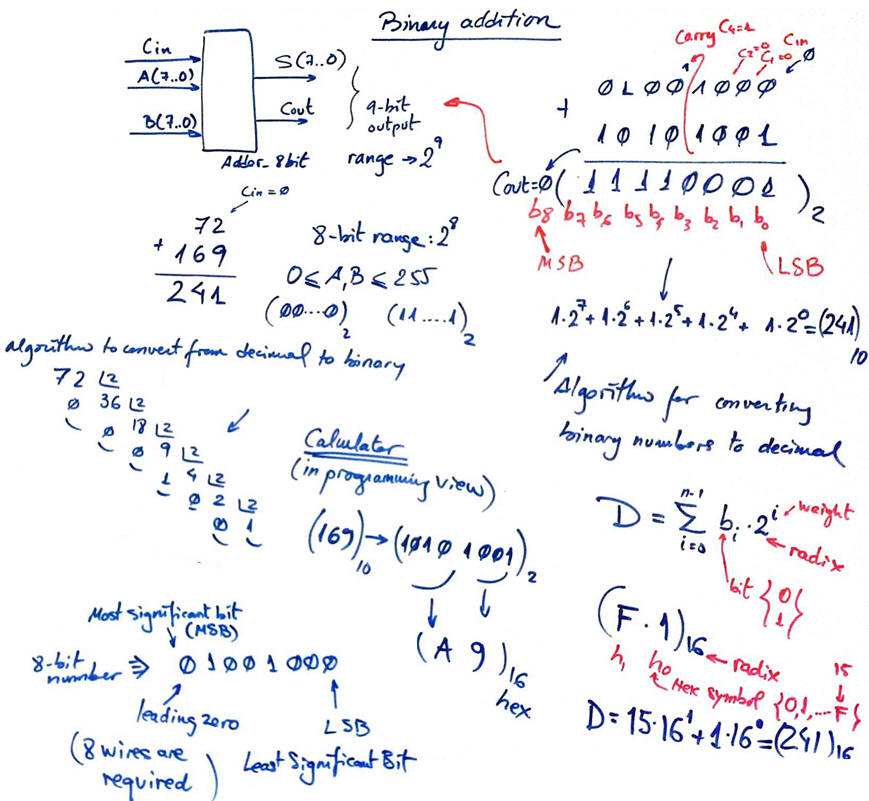Conversion from binary to decimal, decimal to binary. Hexadecimal numbers. How to use the computer calculator in programming mode to work with bin/hex/oct/dec numbers.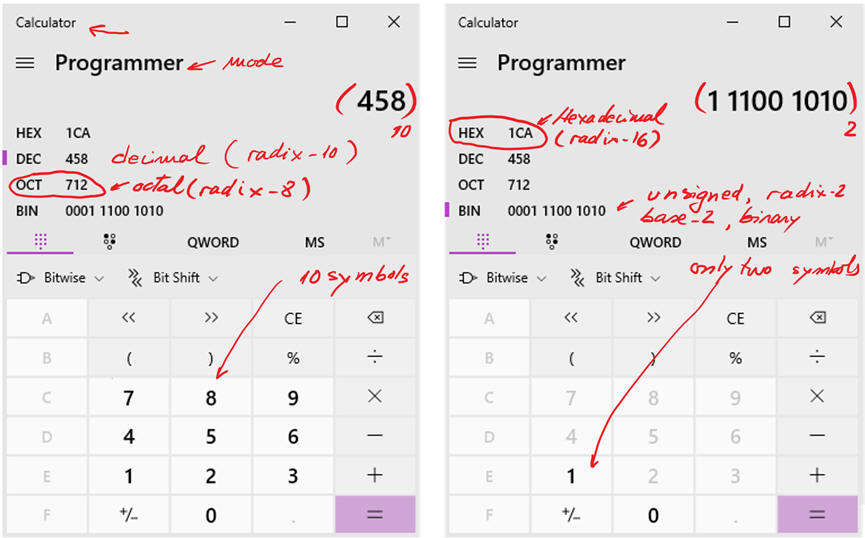Planning 1-bit expandable adders: tutorials on how to design the 1-bit full adder using our plans:

- Plan A: structural (equations)  Adder_1bit

- Plan C2: Hierarchical multiple-file Adder_1bit

1.9.2. Comparator

1.9.2.1. Expandable 1-bit comparator (Comp_1bit)

Symbol and truth table.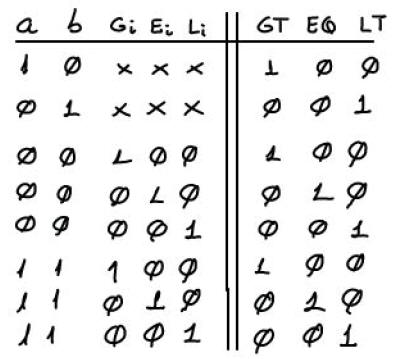Planning expandable comparators:

- Plan A: 1-bit expandable comparator Comp_1bit

- Plan B: 1-bit expandable comparator Comp_1bit

- Plan C2: 1-bit expandable comparator Comp_1bit using the MoM

1.9.3. Multiplier

1.9.3.1. 1-bit multiplier cell, Mult_1bit